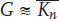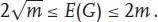## 7.2 Bounds for the Energy of Graphs

Let G be a graph possessing n vertices and m edges. We say that G is an (n, m)-graph.

For any (n, m)-graph ,= 2m.

In what follows we assume that the graph eigenvalues are labeled in a nonincreasing manner, i.e., thatIf G is connected, then λ1 > λ2 . Because λ1 ≥ |λi|, i = 2,..., n, the eigenvalue λ1 is referred to as the spectral radius of graph G.

Some of the simplest and longest standing  bounds for the energy of graphs are given below.

Theorem 7.1  For an (n, m)-graph G,with equality if and only if G is either an empty graph (with m = 0, i.e.,)or a regular graph of degree 1, i.e.,.

Theorem 7.2  For a graph G with m edges,Equality E (G) =holds if and only if G consists of a complete bipartite graph K a,b, such that a· b = m, and ...

Get Analysis of Complex Networks now with the O’Reilly learning platform.

O’Reilly members experience books, live events, courses curated by job role, and more from O’Reilly and nearly 200 top publishers.